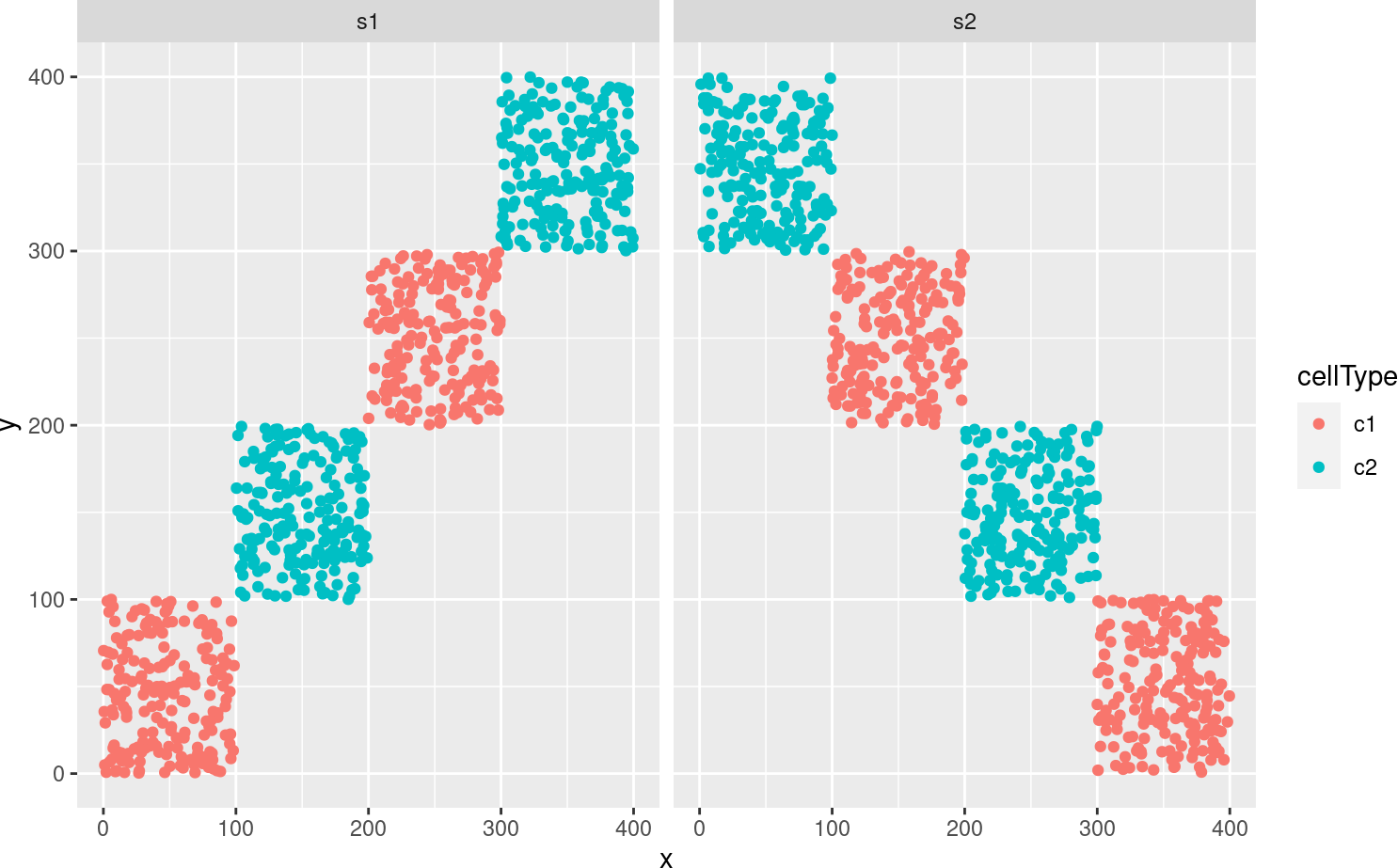# 1 Installation

``````if (!require("BiocManager"))
install.packages("BiocManager")
BiocManager::install("lisaClust")``````
``````# load required packages
library(lisaClust)
library(spicyR)
library(ggplot2)
library(SingleCellExperiment)``````

# 2 Overview

Clustering local indicators of spatial association (LISA) functions is a methodology for identifying consistent spatial organisation of multiple cell-types in an unsupervised way. This can be used to enable the characterization of interactions between multiple cell-types simultaneously and can complement traditional pairwise analysis. In our implementation our LISA curves are a localised summary of an L-function from a Poisson point process model. Our framework `lisaClust` can be used to provide a high-level summary of cell-type colocalization in high-parameter spatial cytometry data, facilitating the identification of distinct tissue compartments or identification of complex cellular microenvironments.

# 3 Quick start

## 3.1 Generate toy data

TO illustrate our `lisaClust` framework, here we consider a very simple toy example where two cell-types are completely separated spatially. We simulate data for two different images.

``````set.seed(51773)
x <- round(c(runif(200),runif(200)+1,runif(200)+2,runif(200)+3,
runif(200)+3,runif(200)+2,runif(200)+1,runif(200)),4)*100
y <- round(c(runif(200),runif(200)+1,runif(200)+2,runif(200)+3,
runif(200),runif(200)+1,runif(200)+2,runif(200)+3),4)*100
cellType <- factor(paste('c',rep(rep(c(1:2),rep(200,2)),4),sep = ''))
imageID <- rep(c('s1', 's2'),c(800,800))

cells <- data.frame(x, y, cellType, imageID)

ggplot(cells, aes(x,y, colour = cellType)) + geom_point() + facet_wrap(~imageID)``````## 3.2 Create SegmentedCellExperiment object

First we store our data in a `SegmentedCells` object.

``````
cellExp <- SegmentedCells(cells, cellTypeString = 'cellType')
``````

## 3.3 Running lisaCLust

We can then use a convience function `lisaClust` to simultaneously calculate local indicators of spatial association (LISA) functions using the `lisa` function and perform k-means clustering.

``cellExp <- lisaClust(cellExp, k = 2)``

## 3.4 Plot identified regions

The `hatchingPlot` function can be used to construct a `ggplot` object where the regions are marked by different hatching patterns. This allows us to plot both regions and cell-types on the same visualization.

``hatchingPlot(cellExp, useImages = c('s1','s2'))``